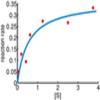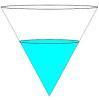Search by Topic

Resources tagged with Cubic functions similar to Curve Fitter:

Filter by: Content type:
Age range:
Challenge level:

There are 7 results

Broad Topics > Functions and Graphs > Cubic functionsCurve Fitter

Age 16 to 18 Challenge Level:

Can you fit a cubic equation to this graph?Cubic Spin

Age 16 to 18 Challenge Level:

Prove that the graph of f(x) = x^3 - 6x^2 +9x +1 has rotational symmetry. Do graphs of all cubics have rotational symmetry?Exploring Cubic Functions

Age 14 to 18 Challenge Level:

Quadratic graphs are very familiar, but what patterns can you explore with cubics?Patterns of Inflection

Age 16 to 18 Challenge Level:

Find the relationship between the locations of points of inflection, maxima and minima of functions.Real(ly) Numbers

Age 16 to 18 Challenge Level:

If x, y and z are real numbers such that: x + y + z = 5 and xy + yz + zx = 3. What is the largest value that any of the numbers can have?Curve Fitter 2

Age 16 to 18 Challenge Level:

Can you construct a cubic equation with a certain distance between its turning points?Fill Me up Too

Age 14 to 16 Challenge Level:

In Fill Me Up we invited you to sketch graphs as vessels are filled with water. Can you work out the equations of the graphs?